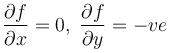Courses

# Partial Derivatives And Euler's Equation MCQ Level - 2

## 10 Questions MCQ Test Topic wise Tests for IIT JAM Physics | Partial Derivatives And Euler's Equation MCQ Level - 2

Description
This mock test of Partial Derivatives And Euler's Equation MCQ Level - 2 for Physics helps you for every Physics entrance exam. This contains 10 Multiple Choice Questions for Physics Partial Derivatives And Euler's Equation MCQ Level - 2 (mcq) to study with solutions a complete question bank. The solved questions answers in this Partial Derivatives And Euler's Equation MCQ Level - 2 quiz give you a good mix of easy questions and tough questions. Physics students definitely take this Partial Derivatives And Euler's Equation MCQ Level - 2 exercise for a better result in the exam. You can find other Partial Derivatives And Euler's Equation MCQ Level - 2 extra questions, long questions & short questions for Physics on EduRev as well by searching above.
QUESTION: 1

### If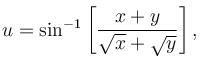then the value of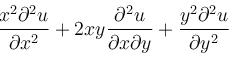is

Solution:

u = sin-1(x + y/(√x + √y))

Put x = xt and y = yt

u = sin-1[(xt-yt)/(√xt + √yt)]

sinu = t1/2[(x+y)/(√x + √y)]

= t1/2 f(x,y)

This function sin u is homogeneous with degree 1/2.

By Euler's theorem

xux + yuy = G(u) = nf(u)/f,(u) = 1/2 tan u

xux + yuy = 1/2 tan u

x2uxx + 2xyuyy + y2uyy = G(u)[G'(u) - 1]

= 1/2 tan u [(sec2u - 2)/2]

= 1/4 tan u [(tan2u - 1)/1]

= 1/4 * sin u/cos u [(sin2u - cos2u)/cos2u]

x2uxx + 2xyuyy + y2uyy = -(sinu cos2u)/4ucos3u

QUESTION: 2

###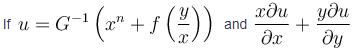then the value of is equal to

Solution:

We have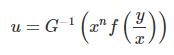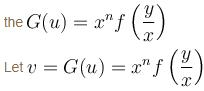v is homogeneous function of degree n then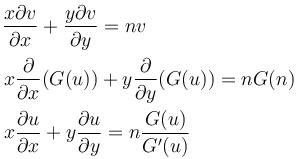The correct answer is: n(n - 1)

QUESTION: 3

### The accompanying figure shows the graph of an unspecified function of f(x, y) and partial derivatives fx(x, y) and fy(x, y). Determine which is which and explain.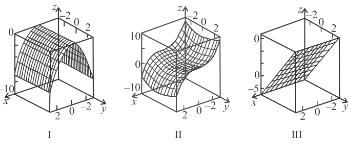Solution:

The correct answer is: II - f(xy),  I - fx(x, y), III - fy(x, y)

QUESTION: 4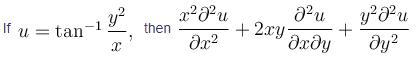Solution:

We are given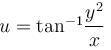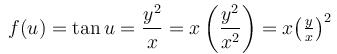f(u) is homogeneous function degree 1, then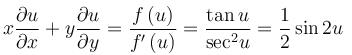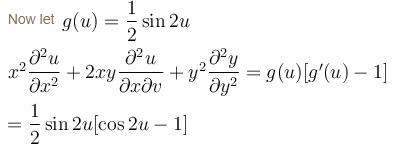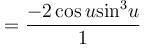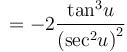[By dividing with cos3 u]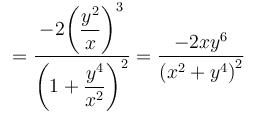The correct answer is: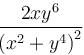QUESTION: 5

If u = f(t) and v = f(t) and  t = φ (x, y)  then

Solution: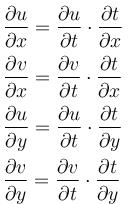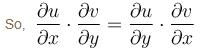The correct answer is: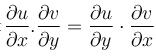QUESTION: 6

If w = f(u, v) where u = x + y and v = x – y then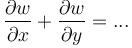Solution:

We have w = f(u, v)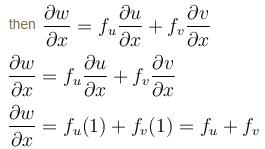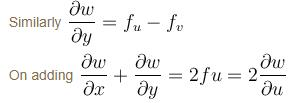The correct answer is: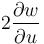QUESTION: 7

If f is differentiable and z = u + f(u2v2), then

Solution:

Let w = u2v2
then z = f(w) + u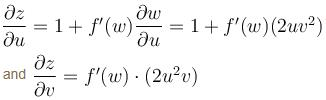So,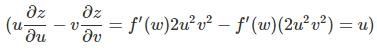The correct answer is: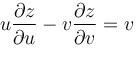QUESTION: 8

If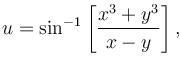then the value of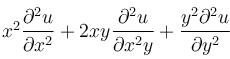is

Solution: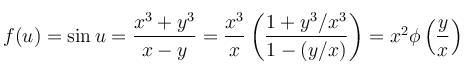f(u) is homogeneous function of degree 2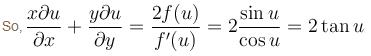Now, let g(u) = 2tan u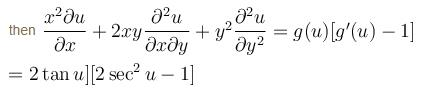The correct answer is: 2tan u(2sec2u –1)

QUESTION: 9

If f(x, y, z) = 0 then the value of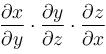equal to

Solution:

(1) Differentiate with respect to y, I get:

0+F2+F3∂z/∂y=0

So

F3 ∂z/∂y = −F2

(2) Differentiate with respect to x, I get:

F1 + F2 ∂y/∂x + 0 = 0

So F2 ∂y/∂x = −F1

(3) Differentiate with respect to z, I get:

F1 ∂x/∂z +0 + F3 = 0

4) After some manipulations with the Fi, I get to the conclusion that

∂z/∂y∗∂y/∂x∗∂x/∂z=−1, so when evaluated with x, z, y respectively, conclusion is still true

QUESTION: 10

Use the information the figure to find the first order partial derivatives of f at the point (1, 2)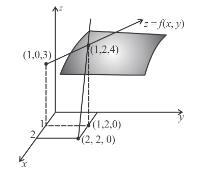Solution:

The correct answer is: